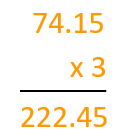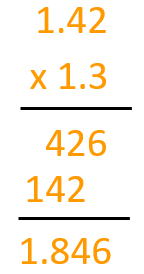Smartick is a fun way to learn math!Dec07

# Multiplication with Decimal Numbers

In this post, we will learn how to perform another operation with decimals: multiplication with decimal numbers.

We are going to look at it case by case:

### Multiplication of decimal numbers with whole numbers:

To multiply a decimal number with a whole number, we multiply the decimal number as if it were a whole number. In the answer, you put the same number of digits after the decimal as there were in the original decimal number.To complete the multiplication of 74.15 x 3, first we multiply as if there are no decimal points, 7415 x 3, and once we finish the multiplication, we notice that 74.15 has two digits after the decimal, so we place a decimal point in such a way that there are decimal places in the answer.

### Multiplication of decimal numbers with decimal numbers:

To multiply a decimal number with a decimal number, we complete the operation as if they were whole numbers. In the answer, you put the same number of digits after the decimal as there were in the two numbers combined.We complete the multiplication as if they were whole numbers: 142 x 13.

Once we finish the multiplication, we have to add the number of decimal places there are in the two original numbers. In this case, 1.42 has two decimal places and 1.3 has one decimal place, making a total of three decimal places, so we place a decimal point in such a way that there are three decimal places in the answer.

### Multiplication of a decimal number by a whole number ending in zeros:

We separate the whole number ending with zeros into a whole number by the following unit from as many zeros as there were previously. Therefore, you multiply the whole number by the decimal number and to finish, you multiply by the number with zeros.

Let’s look at an example so that it becomes clearer. We will multiply 0.04 x 20:

## 0.08 x 10 = 0.8

We separate the whole number followed by zeros into a whole number multiplied by the following unit of zeros, in this case, 20 would be the same as 2 x 10, and therefore the multiplication would be 0.04 x 2 x 10.

We multiply the whole number 2 by the decimal number 0.04.

2 x 0.04 = 0.08

The operation would become 0.08 x 10. To complete this multiplication we only need to move the decimal of 0.08 as many places as there are zeros in the following units, in this case, one place to the right.

Now it’s your turn to practice what you have learned!

Fun is our brain’s favorite way of learning
Diane Ackerman
Smartick is a fun way to learn math
• 15 fun minutes a day